# kmeans

k 均值聚类

## 语法

``idx = kmeans(X,k)``
``idx = kmeans(X,k,Name,Value)``
``[idx,C] = kmeans(___)``
``[idx,C,sumd] = kmeans(___)``
``[idx,C,sumd,D] = kmeans(___)``

## 说明

``idx = kmeans(X,k)` 执行 k 均值聚类，以将 n×p 数据矩阵 `X` 的观测值划分为 `k` 个聚类，并返回包含每个观测值的簇索引的 n×1 向量 (`idx`)。`X` 的行对应于点，列对应于变量。 默认情况下，`kmeans` 使用欧几里德距离平方度量，并用 k-means++ 算法进行簇中心初始化。`

``idx = kmeans(X,k,Name,Value)` 进一步按一个或多个 `Name,Value` 对组参数所指定的附加选项返回簇索引。例如，指定余弦距离、使用新初始值重复聚类的次数或使用并行计算的次数。`

``[idx,C] = kmeans(___)` 在 `k`×p 矩阵 `C` 中返回 `k` 个簇质心的位置。`

``[idx,C,sumd] = kmeans(___)` 在 `k`×1 向量 `sumd` 中返回簇内的点到质心距离的总和。`

``[idx,C,sumd,D] = kmeans(___)` 在 n×`k` 矩阵 `D` 中返回每个点到每个质心的距离。`

## 示例

```load fisheriris X = meas(:,3:4); figure; plot(X(:,1),X(:,2),'k*','MarkerSize',5); title 'Fisher''s Iris Data'; xlabel 'Petal Lengths (cm)'; ylabel 'Petal Widths (cm)';```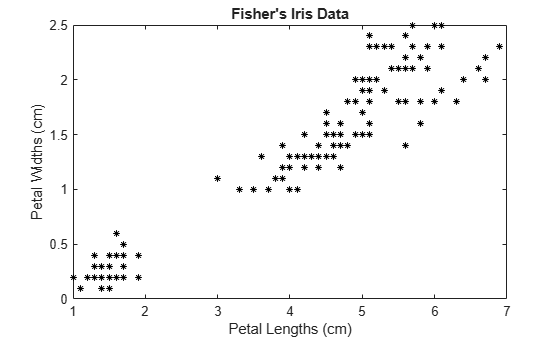```rng(1); % For reproducibility [idx,C] = kmeans(X,3);```

`idx` 是与 `X` 中的观测值对应的预测簇索引的向量。`C` 是包含最终质心位置的 3×2 矩阵。

```x1 = min(X(:,1)):0.01:max(X(:,1)); x2 = min(X(:,2)):0.01:max(X(:,2)); [x1G,x2G] = meshgrid(x1,x2); XGrid = [x1G(:),x2G(:)]; % Defines a fine grid on the plot idx2Region = kmeans(XGrid,3,'MaxIter',1,'Start',C);```
```Warning: Failed to converge in 1 iterations. ```
` % Assigns each node in the grid to the closest centroid`

`kmeans` 显示一条警告，指出算法未收敛，这是您应预料到的，因为软件只实现了一次迭代。

```figure; gscatter(XGrid(:,1),XGrid(:,2),idx2Region,... [0,0.75,0.75;0.75,0,0.75;0.75,0.75,0],'..'); hold on; plot(X(:,1),X(:,2),'k*','MarkerSize',5); title 'Fisher''s Iris Data'; xlabel 'Petal Lengths (cm)'; ylabel 'Petal Widths (cm)'; legend('Region 1','Region 2','Region 3','Data','Location','SouthEast'); hold off;```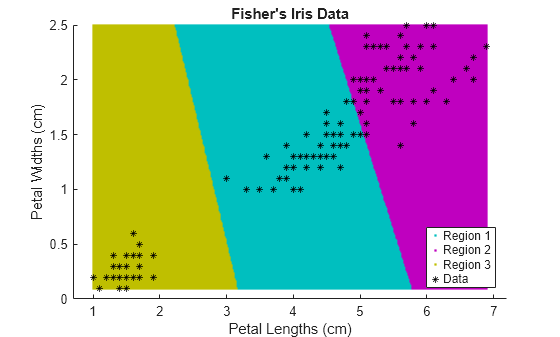```rng default; % For reproducibility X = [randn(100,2)*0.75+ones(100,2); randn(100,2)*0.5-ones(100,2)]; figure; plot(X(:,1),X(:,2),'.'); title 'Randomly Generated Data';```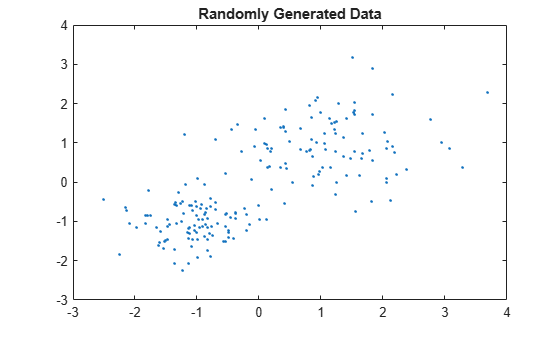```opts = statset('Display','final'); [idx,C] = kmeans(X,2,'Distance','cityblock',... 'Replicates',5,'Options',opts);```
```Replicate 1, 3 iterations, total sum of distances = 201.533. Replicate 2, 5 iterations, total sum of distances = 201.533. Replicate 3, 3 iterations, total sum of distances = 201.533. Replicate 4, 3 iterations, total sum of distances = 201.533. Replicate 5, 2 iterations, total sum of distances = 201.533. Best total sum of distances = 201.533 ```

```figure; plot(X(idx==1,1),X(idx==1,2),'r.','MarkerSize',12) hold on plot(X(idx==2,1),X(idx==2,2),'b.','MarkerSize',12) plot(C(:,1),C(:,2),'kx',... 'MarkerSize',15,'LineWidth',3) legend('Cluster 1','Cluster 2','Centroids',... 'Location','NW') title 'Cluster Assignments and Centroids' hold off```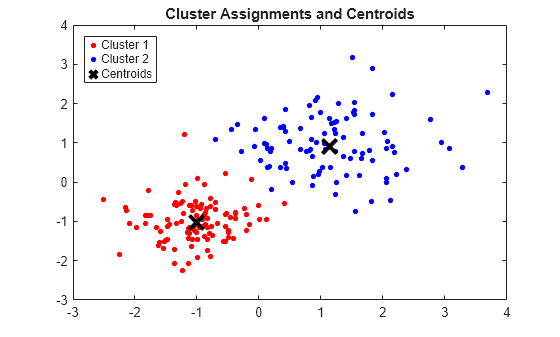```Mu = bsxfun(@times,ones(20,30),(1:20)'); % Gaussian mixture mean rn30 = randn(30,30); Sigma = rn30'*rn30; % Symmetric and positive-definite covariance Mdl = gmdistribution(Mu,Sigma); % Define the Gaussian mixture distribution rng(1); % For reproducibility X = random(Mdl,10000);```

`Mdl` 是一个 30 维 `gmdistribution` 模型，包含 20 个分量。`X` 是一个 10000×30 矩阵，其数据出自 `Mdl` 模型。

```stream = RandStream('mlfg6331_64'); % Random number stream options = statset('UseParallel',1,'UseSubstreams',1,... 'Streams',stream);```

`RandStream` 的输入参数 `'mlfg6331_64'` 指定使用乘法滞后 Fibonacci 生成器算法。`options` 是一个结构体数组，其字段指定控制估算的选项。

```tic; % Start stopwatch timer [idx,C,sumd,D] = kmeans(X,20,'Options',options,'MaxIter',10000,... 'Display','final','Replicates',10);```
```Starting parallel pool (parpool) using the 'local' profile ... connected to 6 workers. Replicate 5, 72 iterations, total sum of distances = 7.73161e+06. Replicate 1, 64 iterations, total sum of distances = 7.72988e+06. Replicate 3, 68 iterations, total sum of distances = 7.72576e+06. Replicate 4, 84 iterations, total sum of distances = 7.72696e+06. Replicate 6, 82 iterations, total sum of distances = 7.73006e+06. Replicate 7, 40 iterations, total sum of distances = 7.73451e+06. Replicate 2, 194 iterations, total sum of distances = 7.72953e+06. Replicate 9, 105 iterations, total sum of distances = 7.72064e+06. Replicate 10, 125 iterations, total sum of distances = 7.72816e+06. Replicate 8, 70 iterations, total sum of distances = 7.73188e+06. Best total sum of distances = 7.72064e+06 ```
`toc % Terminate stopwatch timer`
```Elapsed time is 61.915955 seconds. ```

`kmeans` 执行 k 均值聚类以将数据划分为 k 个簇。当您有要进行聚类的新数据集时，可以使用 `kmeans` 创建包含现有数据和新数据的新簇。`kmeans` 函数支持 C/C++ 代码生成，因此您可以生成接受训练数据并返回聚类结果的代码，然后将代码部署到设备上。在此工作流中，您必须传递训练数据，训练数据有可能相当大。为了节省设备上的内存，您可以分别使用 `kmeans``pdist2` 来分离训练和预测。

```rng('default') % For reproducibility X = [randn(100,2)*0.75+ones(100,2); randn(100,2)*0.5-ones(100,2); randn(100,2)*0.75];```

`[idx,C] = kmeans(X,3);`

```figure gscatter(X(:,1),X(:,2),idx,'bgm') hold on plot(C(:,1),C(:,2),'kx') legend('Cluster 1','Cluster 2','Cluster 3','Cluster Centroid')```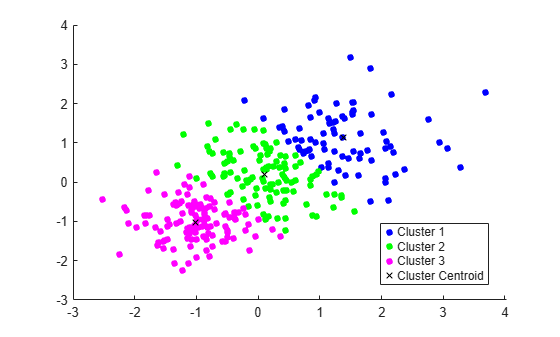```Xtest = [randn(10,2)*0.75+ones(10,2); randn(10,2)*0.5-ones(10,2); randn(10,2)*0.75];```

`[~,idx_test] = pdist2(C,Xtest,'euclidean','Smallest',1);`

```gscatter(Xtest(:,1),Xtest(:,2),idx_test,'bgm','ooo') legend('Cluster 1','Cluster 2','Cluster 3','Cluster Centroid', ... 'Data classified to Cluster 1','Data classified to Cluster 2', ... 'Data classified to Cluster 3')```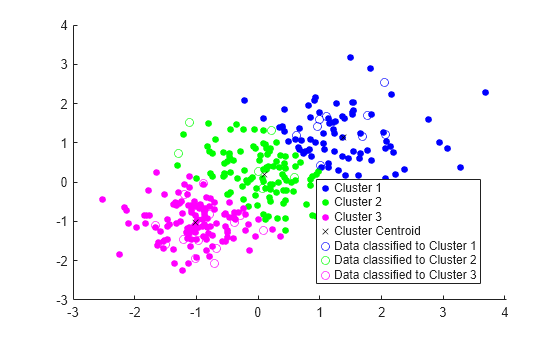`type findNearestCentroid % Display contents of findNearestCentroid.m`
```function idx = findNearestCentroid(C,X) %#codegen [~,idx] = pdist2(C,X,'euclidean','Smallest',1); % Find the nearest centroid ```

`codegen findNearestCentroid -args {C,Xtest}`
```Code generation successful. ```

`codegen` 生成 MEX 函数 `findNearestCentroid_mex`，扩展名因平台而异。

```myIndx = findNearestCentroid(C,Xtest); myIndex_mex = findNearestCentroid_mex(C,Xtest); verifyMEX = isequal(idx_test,myIndx,myIndex_mex)```
```verifyMEX = logical 1 ```

`isequal` 返回逻辑值 1 (`true`)，这意味着所有输入都相等。这一比较结果确认 `pdist2` 函数、`findNearestCentroid` 函数和 MEX 函数均返回相同的索引。

```cfg = coder.gpuConfig('mex'); codegen -config cfg findNearestCentroid -args {C,Xtest} ```

## 输入参数

### 名称-值参数

• `'final'` - 显示最终迭代的结果

• `'iter'` - 显示每次迭代的结果

• `'off'` - 不显示任何内容

`p` 维空间中的距离度量，用于最小化距离和，指定为以逗号分隔的对组，其中包含 `'Distance'``'sqeuclidean'``'cityblock'``'cosine'``'correlation'``'hamming'`

`'sqeuclidean'`

`$d\left(x,c\right)=\left(x-c\right)\left(x-c{\right)}^{\prime }$`

`'cityblock'`

`$d\left(x,c\right)=\sum _{j=1}^{p}|{x}_{j}-{c}_{j}|$`

`'cosine'`

1 减去点之间夹角的余弦值（视为向量）。每个质心是该簇中各点的均值（在将这些点归一化为单位欧几里德长度之后）。

`$d\left(x,c\right)=1-\frac{xc\prime }{\sqrt{\left(x{x}^{\prime }\right)\left(cc\prime \right)}}$`

`'correlation'`

1 减去点之间的样本相关性（视为值序列）。每个质心是该簇中各点的按成分均值（在将这些点中心化并归一化为零均值和单位标准差后）。

`$d\left(x,c\right)=1-\frac{\left(x-\stackrel{\to }{\overline{x}}\right){\left(c-\stackrel{\to }{\overline{c}}\right)}^{\prime }}{\sqrt{\left(x-\stackrel{\to }{\overline{x}}\right){\left(x-\stackrel{\to }{\overline{x}}\right)}^{\prime }}\sqrt{\left(c-\stackrel{\to }{\overline{c}}\right){\left(c-\stackrel{\to }{\overline{c}}\right)}^{\prime }}},$`

• $\stackrel{\to }{\overline{x}}=\frac{1}{p}\left(\sum _{j=1}^{p}{x}_{j}\right){\stackrel{\to }{1}}_{p}$

• $\stackrel{\to }{\overline{c}}=\frac{1}{p}\left(\sum _{j=1}^{p}{c}_{j}\right){\stackrel{\to }{1}}_{p}$

• ${\stackrel{\to }{1}}_{p}$ 是 p 个 1 组成的行向量。

`'hamming'`

`$d\left(x,y\right)=\frac{1}{p}\sum _{j=1}^{p}I\left\{{x}_{j}\ne {y}_{j}\right\},$`

`'error'`

`'drop'`

`'singleton'`

`'Streams'`

`RandStream` 对象或此类对象的元胞数组。如果您没有指定 `Streams``kmeans` 将使用默认流。如果您指定 `Streams`，请使用单个对象，除非满足以下所有条件：

• 您有一个打开的并行池。

• `UseParallel``true`

• `UseSubstreams``false`

`'UseParallel'`
• 如果为 `true``Replicates` > 1，则 `kmeans` 以并行方式对每次重复实现 k 均值算法。

• 如果未安装 Parallel Computing Toolbox，则计算以串行模式进行。默认值为 `false`，表示串行计算。

`'UseSubstreams'`设置为 `true` 将以可重现的方式执行并行计算。默认值为 `false`。要以可重现方式执行计算，请将 `Streams` 设置为允许子流的类型：`'mlfg6331_64'``'mrg32k3a'`

`'cluster'`

`X` 的一个随机 10% 子样本中的观测值数目多于 `k` 个时，对该子样本执行初步聚类阶段。此初步阶段本身是使用 `'sample'` 进行初始化的。

`'plus'`（默认值）实现用于簇中心初始化的 k-means++ 算法，依算法选择 `k` 个种子。
`'sample'``X` 中随机选择 `k` 个观测值。
`'uniform'``X` 范围内随机均匀选择 `k` 个点。Hamming 距离无效。

## 详细信息

### k 均值聚类

k 均值聚类，即 Lloyd 算法 ，是一种迭代数据划分算法，它将 n 个观测值分配给由质心定义的 k 个簇之一，其中 k 是在算法开始之前选择的。

1. 选择 k 个初始簇中心（质心）。例如，随机选择 k 个观测值（通过使用 `'Start','sample'`）或使用 k-means ++ 算法进行簇中心初始化（默认值）。

2. 计算所有观测值到每个质心的点到簇质心的距离。

3. 有两种方法可以继续（由 `OnlinePhase` 指定）：

• 批量更新 - 将每个观测值分配给离质心最近的簇。

• 在线更新 - 只要将观测值重新分配给另一质心可减少簇内点到质心距离平方和的总和，就对该观测值执行此分配。

有关详细信息，请参阅算法

4. 计算每个簇中观测值的平均值，以获得 k 个新质心位置。

5. 重复步骤 2 到 4，直到簇分配不变，或达到最大迭代次数。

### k-means++ 算法

k-means++ 算法使用启发式方法找到 k 均值聚类的质心种子。根据 Arthur 和 Vassilvitskii 的研究·，k-means++ 改进了 Lloyd 算法的运行时间和最终解的质量。

k-means++ 算法按如下方式选择种子，假设簇数为 k。

1. 从数据集 X 中随机均匀选择一个观测值。所选观测值是第一个质心，表示为 c1

2. 计算从每个观测值到 c1 的距离。将 cj 和观测值 m 之间的距离表示为 $d\left({x}_{m},{c}_{j}\right)$

3. 从 X 中随机选择下一个质心 c2，概率为

`$\frac{{d}^{2}\left({x}_{m},{c}_{1}\right)}{\sum _{j=1}^{n}{d}^{2}\left({x}_{j},{c}_{1}\right)}.$`

4. 要选择中心 j，请执行以下操作：

1. 计算从每个观测值到每个质心的距离，并将每个观测值分配给其最近的质心。

2. 对于 m = 1、...、n 和 p = 1、...、j - 1，从 X 中随机选择质心 j，概率为

`$\frac{{d}^{2}\left({x}_{m},{c}_{p}\right)}{\sum _{\left\{h;{x}_{h}\in {C}_{p}\right\}}^{}{d}^{2}\left({x}_{h},{c}_{p}\right)},$`

其中 Cp 是最接近质心 cp 的所有观测值的集合，而 xm 属于 Cp

也就是说，选择每个后续中心时，其选择概率与它到已选最近中心的距离成比例。

5. 重复步骤 4，直到选择了 k 个质心。

Arthur 和 Vassilvitskii· 通过对几个簇方向的模拟研究证明，在计算簇内点到质心距离平方和时，k-means++ 相比 Lloyd 算法能更快地收敛至更低的总和。

## 算法

• `kmeans` 使用两阶段迭代算法来最小化点到质心的距离总和，该距离总和覆盖所有 `k` 个簇。

1. 第一阶段使用批量更新，其中每次迭代都包括一次性将点重新分配给其最近邻的簇质心，然后重新计算簇质心。此阶段有时候不会收敛至局部最小值解。也就是说，将任一点移至不同簇都会增加总距离的数据分区。尤其是对小数据集来说，更容易出现这种情况。批量更新阶段速度很快，但在该阶段逼近的解可能只能作为第二阶段的起点。

2. 第二阶段使用在线更新，即只要单独重新分配点可减少距离的总和，则进行重新分配，并在每次重新分配后重新计算簇质心。此阶段中的每次迭代都对所有点进行一次遍历。此阶段会收敛至一个局部最小值，尽管可能存在总距离更低的其他局部最小值。一般情况下，需要穷尽选择起始点才能求解全局最小值，但是，使用具有随机起始点的几个副本通常也会得到全局最小值解。

• 如果 `Replicates` = r > 1 且 `Start``plus`（默认值），则软件根据 k-means++ 算法选择 r 个可能不同的种子集。

• 如果您在 `Options` 中启用 `UseParallel` 选项且 `Replicates` > 1，则每个工作进程会以并行方式选择种子和进行聚类。

 Arthur, David, and Sergi Vassilvitskii. “K-means++: The Advantages of Careful Seeding.” SODA ‘07: Proceedings of the Eighteenth Annual ACM-SIAM Symposium on Discrete Algorithms. 2007, pp. 1027–1035.

 Lloyd, Stuart P. “Least Squares Quantization in PCM.” IEEE Transactions on Information Theory. Vol. 28, 1982, pp. 129–137.

 Seber, G. A. F. Multivariate Observations. Hoboken, NJ: John Wiley & Sons, Inc., 1984.

 Spath, H. Cluster Dissection and Analysis: Theory, FORTRAN Programs, Examples. Translated by J. Goldschmidt. New York: Halsted Press, 1985.